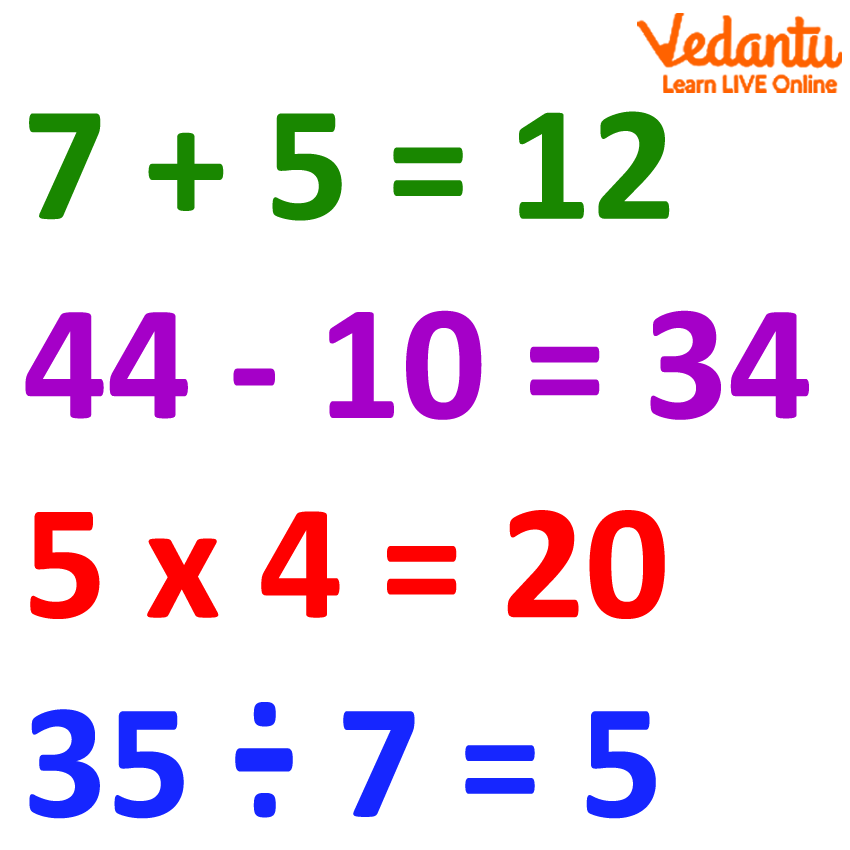Courses
Courses for Kids
Free study material
Free LIVE classes
More

# Number Sentence## What is a Number Sentence in Mathematics?

How to write ten plus ten equals twenty in mathematics? Confused? Don’t be! We are here to help you to form sentences in mathematics. Yes, you heard it right. Sentences in Mathematics!

A number sentence is similar to a normal sentence, which is a collection of subunits that can be used to form proper meaning or sense. In case of a number sentence, numbers and signs are utilised to create mathematical sense. So, number sentences are basically mathematical sentences comprising numbers and mathematical symbols.Let us take a couple of examples to make the concept clear.

5 + 5 = 10

Or

10 + 10 = 20

Here, numbers like 5, 10, and 20 are used in combination with signs like + and = to make a complete sense. If we try to explain this in plain English, we would express it as “Five and five add up to ten” or “Ten and ten add up to twenty”. The structures mentioned above are examples of Equality.

Similarly, we can write mathematical sentences using Inequalities as well. Following are examples of the same:

10 + 5 > 11

Or

15 + 2 < 25

Expressing the above-mentioned sentences in English would include terms like ‘greater than’ and ‘less than’. “The sum of ten and five is greater than eleven” and “The sum of fifteen and two is less than twenty-five”.

## Types of Number Sentences

• Addition Sentences - Mathematical sentences that include the addition operation are termed addition sentences. For instance, 5 + 2 = 7.

• Subtraction Sentences - Mathematical sentences including the subtraction operation are called subtraction sentences. For example, 5 - 2 = 3.

• Multiplication Sentences - Mathematical sentences including the multiplication operation are called multiplication sentences. For example, 5 x 2 = 10.

• Division Sentences - Mathematical sentences including the division operation are called division sentences. For example, 10 ÷ 2 = 5.

• Less Than sentences - Mathematical sentences consisting of the ‘less than’ symbol are called less than sentences. For example, 5 + 2 < 10.

• Greater Than Sentences - Mathematical sentences consisting of the ‘greater than’ symbol are called greater than sentences. For example, 5 + 2 > 6.

• Algebraic Sentences - Mathematical sentences that comprise variables and algebraic components are called algebraic sentences. For example, a + b = c.

• Fraction Sentences - Mathematical sentences including fractions are called fraction sentences. For example, 1/5 + 2/5 = 3/5.

## Points to Remember while Dealing with Number Sentences

1. Number sentences may or may not always be true. For instance, 10 + 2 = 12 is informative and is also true. However, 10 + 2 = 5 is also informative, but it is not true. This number sentence is understandable, but the information it gives is incorrect. It must be noted, however, that wrong number sentences also convey information that can be used to turn the sentence into a correct one. For example, in the equation 10 + 2 = 5 shows the purpose of the operation to be performed. So, by simply changing the value 5, the equation can be turned into a correct number sentence.

2. Number sentences consist of numbers, symbols, operations, and a number after the decisive symbol (equality or inequality). However, if any of these components goes missing, the sentence construction will fail to convey meaning. For example, 10 + 5 > 12 can be deciphered as “the sum of ten and five is greater than twelve”, but the expressions 10 + 5, 10 + 5 >, 10 + 5 12, etc., alone do not make enough sense to be considered a proper number sentence.

3. Number sentences can be in the form of a word problem. For instance, let us consider the following problem:

Katlyn has 8 oranges. Her friend, Ronald, gave her 10 more oranges. What is the total number of oranges Katlyn has now?

Here, to express the problem mathematically, we can say that

Total number of oranges = 8 +  10

= 18

This is how a word problem can be converted into a number sentence that makes perfect sense.

## Conclusion

Number sentences can be simply defined as mathematical expressions for regular sentences that consist of numerical values, mathematical symbols, and operations. These sentences may or may not be correct. However, they serve the purpose of informing, and such information can be used to construct proper, meaningful expressions in mathematics.

Last updated date: 29th Sep 2023
Total views: 141.6k
Views today: 2.41k

## FAQs on Number Sentence

1. Can number sentences be used in daily life?

Number sentences can be used in day to day lives, but they must be expressed using words and phrases to convey easily comprehensible information. In writing, however, number sentences can very much be used. For example, when billing a person at a restaurant, number sentences can be utilised to make the break-up of the bill convenient to read through.

2. How can fraction sentences be used in daily life?

Fraction sentences can make shopping for groceries an easy task. Very often, we are required to buy only a part or portion of an item, not the entire item. For instance, half a watermelon, half a kilogram of salt, and so on. Using fraction sentences makes the job easy as they are traditionally used in market places.